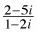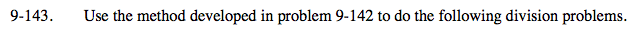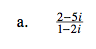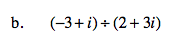Home > A2C > Chapter 9 > Lesson 9.3.2 > Problem9-143

9-143.
1. Use the method developed in problem 9-142 to do the following division problems. Homework Help ✎

1.2. (−3 + i) ÷ (2 + 3i)Use by the conjugate to rationalize the denominator.

$\frac{(2-5\textit{i})(1+2\textit{i})}{(1-2\textit{i})(1+2\textit{i})}$

$\frac{12}{5}-\frac{1}{5}\textit{i}$See part (a).